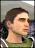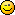Discussion about math, puzzles, games and fun.   Useful symbols: ÷ × ½ √ ∞ ≠ ≤ ≥ ≈ ⇒ ± ∈ Δ θ ∴ ∑ ∫  π  -¹ ² ³ °

You are not logged in.

## #1 2005-09-12 10:16:17

Josh
Guest

### Calculus problem

Well, it's the beginning of the year and I'm already stumped :-X.

The problem tells me to add, subtract, multiply and divide two given functions, but I am not sure how to continue since they are square roots.

Here are the functions:

f(x) = sq.root(1+x) and g(x) = sq.root(1-x)

Find: f+g, f-g, fg, and f/g

Any help is much appreciated.## #2 2005-09-13 07:54:05

Flowers4Carlos
MemberRegistered: 2005-08-25
Posts: 106

### Re: Calculus problem

hi yaz josh!!!

i'm going to take a wild guess and say this is problem #32... am i right???f + g = (1+x)^(1/2) + (1-x)^(1/2)
that's it!!!!  why??  we cannot add radicals.

f - g = (1+x)^(1/2) - (1-x)^(1/2)
that's it!!!  why??  we cannot subtract radicals.

fg = (1+x)^(1/2) * (1-x)^(1/2)
use the property (ab)^(1/2) = (a)^(1/2) * (b)^(1/2)
[(1+x)(1-x)]^(1/2)
(1-x^2)^(1/2)

f/g = (1+x)^(1/2) / (1-x)^(1/2)
use the property: (a/b)^(1/2) = (a)^(1/2) / (b)^(1/2)
[(1+x) / (1-x)]^(1/2)

that's it buddy!!

Offline